# ROW_NUMBER Function in Oracle

## Row_Number Function in Oracle with Examples

In this article, I am going to discuss the Row_Number Function in Oracle with Examples. Please read our previous article where we give an overview of Analytical Functions in Oracle. At the end of this article, you will understand what exactly the ROW_NUMBER function is and when and how to use the Row Number Function in Oracle with real-time examples.

##### ROW_NUMBER Function in Oracle:

The ROW_NUMBER function in Oracle is an analytic function or you can say ranking function that is used when we want to return a unique sequential number starting from 1 for record in the result set. The ROW_NUMBER() function is a built-in function in Oracle that will assign a unique sequential integer number to each row starting from 1 within a partition of a result set. The row number always starts with 1 for the first row in each partition and then increases by 1 for the next row onwards for each partition. The syntax to use the ROW_NUMBER function in Oracle is given below.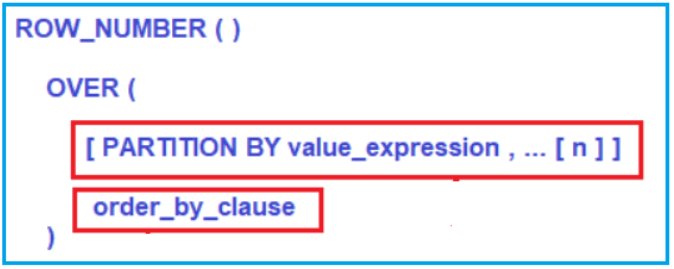###### Parameters:
1. PARTITION BY value_expression: This is optional. The PARTITION BY clause is used to partition the result set that is produced by the FROM clause and then the ROW_NUMBER function is applied to each partition. Here, the value_expression specifies the column name (s) using which the result set is going to be partitioned. As it is optional, if you did not specify the PARTITION BY clause, then the ROW_NUMBER function will treat all the rows of the query as a single partition.
2. ORDER_BY_CLAUSE: This is Mandatory. The ORDER BY clause is used to define the sequence in which each row is going to assign its unique ROW_NUMBER.
##### Examples to Understand ROW_NUMBER Function in Oracle

Let us understand the ROW_NUMBER function in Oracle with examples. We are going to use the following Employees table to understand the ROW_NUMBER function.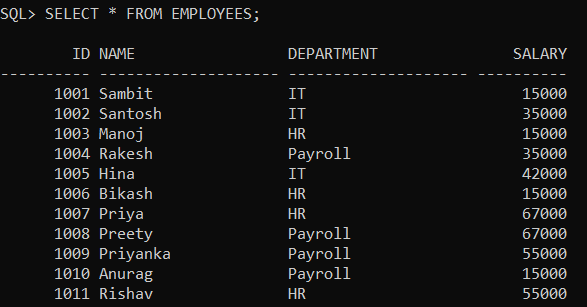Please use the following SQL Script to create and populate the Employees table with the required sample data.

```CREATE TABLE Employees
(
ID INT PRIMARY KEY,
Name VARCHAR2(20),
Department VARCHAR(20),
Salary int
);

INSERT INTO Employees Values (1001, 'Sambit', 'IT', 15000);
INSERT INTO Employees Values (1002, 'Santosh', 'IT', 35000);
INSERT INTO Employees Values (1003, 'Manoj', 'HR', 15000);
INSERT INTO Employees Values (1004, 'Rakesh', 'Payroll', 35000);
INSERT INTO Employees Values (1005, 'Hina', 'IT', 42000);
INSERT INTO Employees Values (1006, 'Bikash', 'HR', 15000);
INSERT INTO Employees Values (1007, 'Priya', 'HR', 67000);
INSERT INTO Employees Values (1008, 'Preety', 'Payroll', 67000);
INSERT INTO Employees Values (1009, 'Priyanka', 'Payroll', 55000);
INSERT INTO Employees Values (1010, 'Anurag', 'Payroll', 15000);
INSERT INTO Employees Values (1011, 'Rishav', 'HR', 55000);
```
##### ROW_NUMBER Function without PARTITION BY Clause in Oracle:

Let us first see an example of the ROW NUMBER function without using the PARTITION BY Clause in Oracle. As we already discussed, if we did not specify the PARTITION BY Clause in Oracle, then the ROW_NUMBER function will treat the whole result set as a single partition. As a result, the ROW_NUMBER function will provide a consecutive sequence numbering for all the rows present in the result set based on the column(s) specified in the order by clause.

For a better understanding, please have a look at the below example where we used the ROW_NUMBER function without using the PARTITION BY clause.

```SELECT Id, Name, Department, Salary,
ROW_NUMBER() OVER (ORDER BY Department) AS RowNumber
FROM Employees;
```

Once you execute the above query, you will get the following output. If you observe the below output, then you can see that there is no partition and hence all the rows are assigned with consecutive sequence numbering starting from 1 to 12 based on the Department column.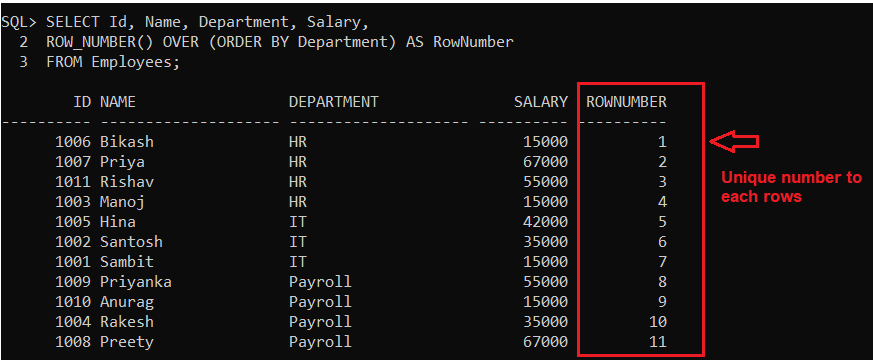##### Row_Number Function with PARTITION BY Clause in Oracle:

Let us see an example of the ROW_NUMBER function with PARTITION BY Clause in Oracle. When we specify the PARTITION BY Clause, then the data is going to be partitioned based on the column we specify in the Partition by clause. Please have a look at the following example code to understand this better. As you can see, here, we have specified Department in the Partition By clause and Name in the Order By clause.

```SELECT Id, Name, Department, Salary,
ROW_NUMBER() OVER
(
PARTITION BY Department
ORDER BY Name
) AS RowNumber
FROM Employees;
```

When you execute the above query, you will get the following output. In our Employees table, we have three departments. So, the Partition by Clause will divide all the rows into three partitions (IT, HR, and Payroll). Then on each partition, the data is sorted based on the Name column. Finally, the ROW_NUMBER function gives a unique sequence integer number starting from 1 to each partition. As we have three partitions, each partition will start from 1, and that you can see in the below image.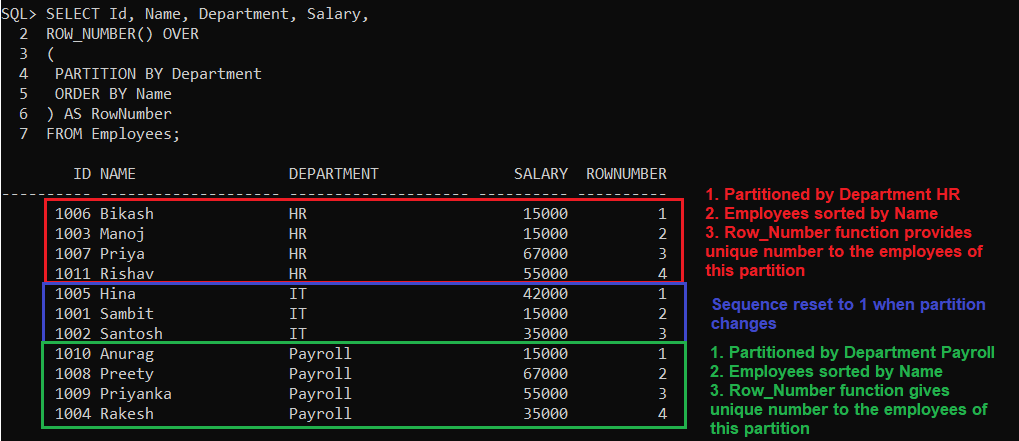##### Finding top N rows of every group using Row Number function in Oracle

To understand how to find the top n rows of every group we are going to use the same Employees table. As you can see, the Employees table contains three department employees. We can use the ROW_NUMBER() function to find the top N rows for every group. For example, let us see how to find the top two employees of each department based on the highest salary. The following SQL query uses the ROW_NUMBER() function to find the top two employees of each department based on the highest salary.

```WITH HighestSalaryEmployees
AS
(
SELECT Id, Name, Department, Salary,
ROW_NUMBER() OVER
(
PARTITION BY Department
ORDER BY Salary DESC
) RowNumber
FROM Employees
)
SELECT Id, Name, Department, Salary
FROM HighestSalaryEmployees
WHERE RowNumber <= 2;
```

Once you execute the above query, you will get the following desired output.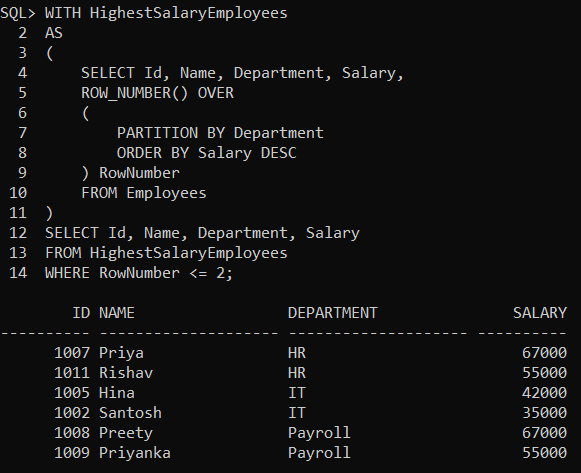##### Pagination using ROW_NUMBER() Function in Oracle

As the ROW_NUMBER() function in Oracle assigns a unique integer number to each row in the result, we can use it for pagination. For example, we need to display a list of employees with 5 employees per page. To get all the employees for the second page, we need to use the following SQL query.

```SELECT * FROM
(
SELECT Id, Name, Department, Salary,
ROW_NUMBER() OVER (ORDER BY Id) AS RowNumber
FROM Employees
) temp
WHERE RowNumber BETWEEN 6 AND 10;
```

Once you execute the above query, you will get the following desired output.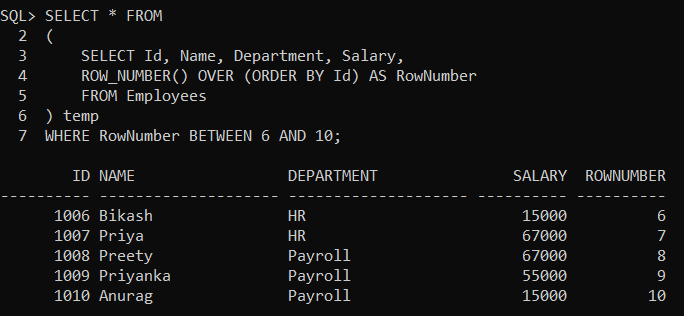##### Deleting Duplicate Records in Oracle using ROW_NUMBER Function:

To understand this, we are going to use the following Employee table. Here, intentionally we have inserted some duplicate data into the Employee table.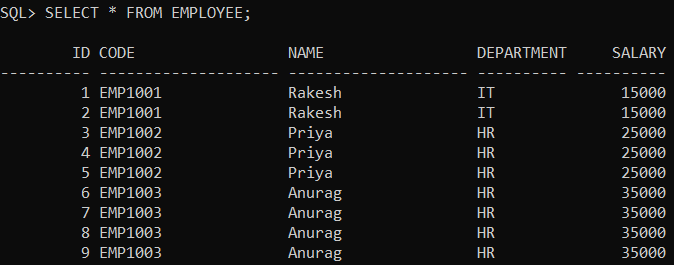Please use the following SQL Script to create the Employee table as well as populate the Employee table with the required sample data.

```CREATE TABLE Employee
(
ID INT PRIMARY KEY,
Code VARCHAR(20),
Name VARCHAR(20),
Department VARCHAR2(10),
Salary int
);

INSERT INTO Employee Values (1, 'EMP1001', 'Rakesh', 'IT', 15000);
INSERT INTO Employee Values (2, 'EMP1001', 'Rakesh', 'IT', 15000);
INSERT INTO Employee Values (3, 'EMP1002', 'Priya', 'HR', 25000);
INSERT INTO Employee Values (4, 'EMP1002', 'Priya', 'HR', 25000);
INSERT INTO Employee Values (5, 'EMP1002', 'Priya', 'HR', 25000);
INSERT INTO Employee Values (6, 'EMP1003', 'Anurag', 'HR', 35000);
INSERT INTO Employee Values (7, 'EMP1003', 'Anurag', 'HR', 35000);
INSERT INTO Employee Values (8, 'EMP1003', 'Anurag', 'HR', 35000);
INSERT INTO Employee Values (9, 'EMP1003', 'Anurag', 'HR', 35000);
```

Now we need to delete the duplicate records from the Employee table. Once we delete the duplicate records, then our Employee table should have the following three records.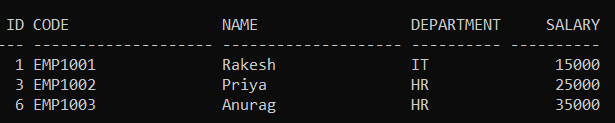The following SQL query will delete the duplicate records from the Employee table. Here, the PARTITION BY clause divides the result set into partitions based on the Employee Code column. That means it will create multiple partitions based on the Employee Code column value and give sequential numbers starting from 1 for each partition. Then the DELETE statements will delete the records from each partition where the RowNumber is greater than 1. It means it will keep only one record from each partition and delete the rest of all.

```DELETE FROM EMPLOYEE WHERE ROWID IN
(
SELECT RWID FROM (SELECT ROWID RWID,
ROW_NUMBER() OVER ( PARTITION BY CODE ORDER BY ID) ROWNUMBER
FROM   EMPLOYEE )
WHERE  ROWNUMBER>1
);
```

When you will execute the above query, you will get the following message saying 6 rows deleted.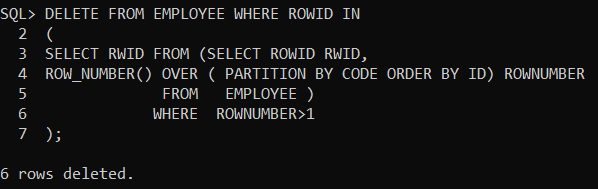In the next article, I am going to discuss the RANK Function in Oracle with examples. Here, in this article, I try to explain the need and use of the ROW NUMBER Function in Oracle with Examples. I hope you enjoy this ROW NUMBER function article.